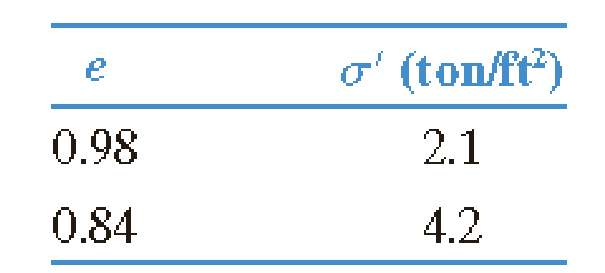Chapter 11, Problem 11.12PPrinciples of Geotechnical Enginee...

9th Edition
Braja M. Das + 1 other
ISBN: 9781305970939

Solutions

Chapter
SectionPrinciples of Geotechnical Enginee...

9th Edition
Braja M. Das + 1 other
ISBN: 9781305970939
Textbook Problem

An undisturbed clay has a preconsolidation pressure of 1.9 ton/ft2 at a void ratio of 1.1. Laboratory consolidation test yields the following data:a. Determine the void ratio that corresponds to a pressure 6 ton/ft2. b. What would be the void ratio at a pressure of 1.5 ton/ft2? Given: C s = 1 5 C c .

(a)

To determine

Calculate the void ratio that corresponds to a pressure 6ton/ft2.

Explanation

Given information:

The preconsolidation pressure (σc) is 1.9ton/ft2.

The void ratio of clay (e) is 1.1.

The void ratio (e1) is 0.98.

The void ratio (e2) is 0.84.

The pressure (σ1) is 2.1ton/ft2.

The pressure (σ2) is 4.2ton/ft2.

The pressure (σ3) is 6ton/ft2.

Calculation:

Calculate the compression index (CC) using the relation.

CC=e1e2log(σ2σ1)

Substitute 0.98 for e1, 0.84 for e2, 2.1ton/ft2 for σ1, and 4

(b)

To determine

Calculate the void ratio at a pressure of 1.5ton/ft2.

Still sussing out bartleby?

Check out a sample textbook solution.

See a sample solution

The Solution to Your Study Problems

Bartleby provides explanations to thousands of textbook problems written by our experts, many with advanced degrees!

Get Started

For Problem 19.39, calculate the probability distribution and plot the probability distribution curve.

Engineering Fundamentals: An Introduction to Engineering (MindTap Course List)

List three methods of applying lubricants to machine tools.

Precision Machining Technology (MindTap Course List)

What kind of ticket is held by Kerbeross TGS?

Network+ Guide to Networks (MindTap Course List)

What emergency steps should be taken to treat burns?

Welding: Principles and Applications (MindTap Course List)